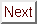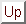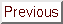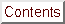Next: Discussion and Conclusions Up: Possible Interpretations of Previous: Critical Field Enhancement

## Multiband and Anisotropic Gap Models of Superconductivity

There are two-types of models which take anisotropy as a point of departure, rather than treating it as a small perturbation, as the pioneering Hohenberg and Werthamer model did. One class consists of models that consider a single non-spherical Fermi surface and/or energy gap. This category includes work by Takanaka, Pohl and Teichler, Youngner and Klemm, Butler, and Prohammer and Schachinger. The other class of theories study the implications of two different Fermi surface pieces that contribute to superconductivity. These models have been developed by Entel and Peter,[80,81] Decroux,, and Al-Jishi.

Theories in the first class, consisting of the anisotropic gap/Fermi surface models, are essentially extensions of the AGL model discussed previously. That is, these theories contain the essential physics of the AGL model plus modifications describing what are called non-local effects. ``Non-local'' is an adjective that refers to clean-limit phenomena outside the the scope of Ginzburg-Landau theories which are related to the finite spatial extent of the Cooper pair. That the more complex models reduce to the AGL model in the local limit is demonstrated by Hohenberg and Werthamer. What Hohenberg and Werthamer did was to rewrite the eigenvalue equation for the energy gap (which is an intermediate step in the derivation of the Helfand-Werthamer equation for Hc2) in terms of an infinite sum. The sum has terms which are matrix elements of even powers of a quantity proportional to vecv cdot vecpi, where vecv is the Fermi velocity and vecpi == hbar/i vecnabla - e/c vecA is the gauge-invariant momentum operator. Because of the relationship between the vector potential vecA and the magnetic field, it turns out that the component of vecv parallel to vecpi is orthogonal to the applied field, in keeping with the expectation from the GL theory that transport in a plane perpendicular to the applied field determines Hc2. The important quantity in this formalism iswhere the integral is to be taken over the FS. Here vecq is a point on the Fermi surface, N(vecq) is the density of states at that point, and v_|_ ^H (vecq) is the component of vecvF(vecq) perpendicular to vecH. Obviously the critical field anisotropy is uniquely specified by one parameter epsilon only if the FS is ellipsoidal. Otherwise knowledge of the entire FS geometry is needed. Notice that v_|_ vecH (vecq) depends not only on the magnitude of vecvF at the point vecq, but also on the angle between the angle between vecH and vecvF(vecq). This point is illustrated by Fig.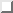from Ref. .Figure: Illustration of how v_|_ vecH (vecq) changes as a function of wavevector vecq for an ellipsoidal Fermi surface. vecq is the coordinate of a point on the Fermi surface. B is Dalrymple's anisotropy parameter, which is equivalent to epsilon in the AGL model.

Hohenberg and Werthamer went on to show that keeping only the zeroth-order term in the infinite sum gives the isotropic theory, while keeping terms up to the second order in the dot product gives the AGL model, including Eqn.for Hc2(theta). What all subsequent models of FS/gap anisotropy do is to include more terms in the sum over powers of ( vecv cdot vecpi). Increasingly higher-order terms correspond both to higher degrees of non-locality, and because of symmetry, also to more complex FS/gap geometries ( i.e., higher angular momentum spherical harmonics). Hohenberg and Werthamer included fourth-order contributions perturbatively, which corresponds to the weak-anisotropy limit. The weak-anisotropy limit is probably less appropriate for GIC's than for superconductors like niobium or vanadium, which have 1/epsilon on the order of 2 or 3. The Hohenberg and Werthamer model only modifies Hc2(t) near Tc, and cannot produce the low-t enhancement observed in GIC's.

Takanaka included terms up to order four in a non-perturbative manner, but his theory is limited to t 1 because of an error.[274,114] Youngner and Klemm, who fit their equations to NbSe2 data, found a way to sum the series exactly up to infinite order. They remark that their model is basically equivalent to that of Pohl and Teichler, who fit data on vanadium. These models are also very similar to the work of Butler, who applied his theory to niobium, except that Butler dropped all terms due to gap anisotropy in his final equations because he thought were quite small. Butler's equations have also been used by Dalrymple and Prober to fit data on Nb1-xTaxSe2.

From examination of the h* versus t curves that appear in either Butler's paper or Youngner and Klemm's paper, it is clear that either one can produce both extended linearity using an ellipsoidal Fermi surface model. This is encouraging because the pi-bands of GIC's are generally believed to produce an ellipsoidal FS piece, but it is not the whole story. A calculation using Butler's equations taken from Dalrymple's thesis is shown in Fig.. Notice that h*(t) is enhanced only when Dalrymple's parameter B (which is the same for an ellipsoidal Fermi surface as epsilon from the AGL model) is less than one, and that h*(0) is actually suppressed from the isotropic value when B is greater than one. The significance of this conclusion is that for a simple ellipsoidal FS, the Butler model extended linearity gives only for one field orientation. Therefore one cannot describe critical field enhancement for both field orientations using a simple ellipsoidal FS.Figure: Enhanced linearity of h*(t) calculated from Butler's equations using an ellipsoidal FS model. Taken from Ref. . Dalrymple's parameter B is equivalent to 1/epsilon in the AGL model. The B = 1.0 curve is for a spherical Fermi Surface, and so is equivalent to the WHH theory.

Because Dalrymple and Prober observed enhanced critical fields for NbSe2 for both vecH || ^c and vecH _|_ ^c, they tried fitting their data with more complex Fermi surface geometries. When they combined an ellipsoid with a cylindrical FS model calculated by Wexler and Woolley, they found excellent quantitative agreement between theory and experiment for vecH || ^c, and good qualitative agreement for vecH _|_ ^c. These fits are shown in Fig.. In order to get quantitative agreement for vecH _|_ ^c, the authors had to multiply all the data through by a factor of 2.1. Dalrymple and Prober explained that this factor was necessary because of mean-free-path anisotropy, which is not taken into account in the Butler model. The mean-free path l comes in because of the dependence of Hc2 on the diffusivity D == 1/3 vF l. This type of mean-free-path anisotropy is quite believable since much larger ratios have often been seen in GIC's. Essentially Dalrymple and Prober find that the Butler model describes Hc2, || ^c data without correction. This agreement occurs since in-plane transport in NbSe2 is clean, and Butler's model is for clean-limit superconductors. The Butler model needs a correction to fit Hc2, _|_ ^c data because transport along ^c is dirty.Figure: Butler-model fit of NbSe2 Hc2(t) data. Figures taken from Dalrymple's thesis. a) Hc2, _|_ = Hc2, || ^c. An excellent fit is obtained by using the Wexler-Woolley Fermi Surface model plus an additional ellipsoid. b) Hc2, || = Hc2, _|_ ^c. The Wexler-Woolley-plus-ellipsoid model produces the correct shape, but needs to be multiplied by an additional factor of 2.1 to account for mean-free-path anisotropy.

Youngner and Klemm's model of Hc2(T) seems to be the same as Butler's, but with the added feature of gap anisotropy. In this case, vecv(vecq) cdot vecpi may still depend on vecq. In addition, though, the states between which the matrix element of vecv cdot vecpi is evaluated may also themselves be non-spherical. Youngner's model produces curves quite similar to those of Butler, including extended linearity and positive curvature for some choices of parameters. The recent theory of Prohammer and Schachinger is nearly the same as Youngner and Klemm's model, only these authors consider electron-phonon coupling anisotropy rather than explicit gap anisotropy.

As if these weren't already enough models, there are also theories which consider the effect of multiple bands contributing to superconductivity. The most extensive of these was developed by Entel and Peter. A two-band model fit by Entel and Peter to data on Cs0.1WO2.9F0.1, a tungsten fluoroxide bronze, is shown in Fig.. Decroux and Fischer advocate the use of the Entel-Peter model for fits to ternary molybdenum chalcogenide data. The main parameters which determine the critical field enhancement in this theory are the interband and intraband scattering times. Al-Jishi has proposed a theory quite similar to Entel and Peter's, but his critical field calculations are still in a preliminary phase. In GIC terms, these models propose that intercalant and graphitic bands both contribute to superconductivity. This idea was originally suggested by Al-Jishi, and it is supported by a large amount of experimental evidence, as is described in Section.Figure: Two-band model fit to anomalous Hc2(t) of Cs0.1WO2.9F0.1 from Ref. . The plot is of h* versus t. The curve labeled (4) is the Helfand-Werthamer isotropic theory. The crosses, circles and squares are experimental data for three different crystallographic orientations (the orientations are not specified). Curve (1) is the two-band model with no interband-scattering, whereas (2) and (3) correspond to increasing interband-scattering. The parameters of these fits are too numerous to list here, but may be found in Ref. .

The profusion of models which predict an enhanced Hc2(T) is quite confusing, especially since both the multiband and anisotropic gap/FS theories seem to have features which are very sensible for GIC's. Prohammer and Schachinger say in their recent paper that the Entel-Peter model and the anisotropic gap/FS models are actually equivalent, really amounting to different parameterizations of the same phenomena. Considering the similarities of the curves in Figs.and, this is not too surprising.

The question of whether these models will explain the anomalies in GIC Hc2(T) data, ranging from the extended linearity of C4KHg to the positive curvature of C8RbHg, still has not been directly addressed. Clearly all of the multiband and anisotropic gap/FS theories are capable of producing curves of the right shape, but this doesn't mean that they would give good fits to the data using reasonable FS/gap parameters. Unfortunately, no complete theoretical Fermi surface computation for the ternary GIC's is available, and there is only limited knowledge about the normal-state transport properties. Holzwarth has calculated the band structure of C4KHg, but published only a qualitative sketch of the Fermi surface. This sketch is reproduced in Fig.. If Holzwarth publishes quantitative information about the FS of C4KHg, or, even better, FS information for C8RbHg, then a fit could be made to the critical field data. Obviously this hypothetical fit would be the decisive test of the applicability of these models.Figure: Fermi surface computed for C4KHg by Holzwarth and colleagues. The basic structure of the Fermi surface is similar to that of NbSe2 in that both have pieces of nearly cylindrical symmetry at the corner of a hexagonal Brillouin zone, and both have higher masses for transport along kz than in the layer planes. The hexagonal solid line is the Brillouin zone; the roughly triangular pieces drawn with a solid line at the corners of the BZ are the graphitic pi bands. The pieces drawn with a dotted line are due to mercury bands. The small circular zone-center part is from Hg 6s holes; the hexagonal portion is from Hg 6ppi electron carriers; and the trigonal pieces at the zone corner are derived from H 6psigma bands.

Lamentably an attempt to invert the critical field data to predict the geometry of the Fermi surface is not justified by the critical field data which is available. In principle at least, if Hc2(T) had been measured at a large number of angles theta, this inversion could be performed.

What conclusions can be drawn about the appropriateness of the anisotropic gap/FS or multiband models for GIC's in the absence of a fit? On the plus side, these models contain features compatible with what is already known about GIC's: GIC's have highly anisotropic Fermi surfaces; they are more disordered along the c-axis than in-plane; they have multiple bands present at the Fermi surface; and their h*(t) curves are much like those of the transition metal dichalcogenides for whom most of the models were intended. Therefore the tentative conclusion is that GIC superconductivity is described by one of these theories, although for positive confirmation, fits to the data are still needed.Next: Discussion and Conclusions Up: Possible Interpretations of Previous: Critical Field Enhancement

alchaiken@gmail.com (Alison Chaiken)
Wed Oct 11 22:59:57 PDT 1995